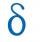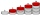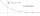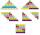# Common difference

The 4th term of an arithmetic progression is 6. If the sum of the 8th and 9th terms is -72, find the common difference.

d =  -9.3333

### Step-by-step explanation:Did you find an error or inaccuracy? Feel free to write us. Thank you!## Related math problems and questions:

• Arithmetic progression 2The 3rd term of an Arithmetic progression is ten more than the first term, while the fifth term is 15 more than the second term. Find the sum of the 8th and 15th terms of the Arithmetic progression if the 7th term is seven times the first term.
• Geometric progressiobIf the sum of four consective terms of geometric progression is 80 and arithmetic mean of second and fourth term is 30 then find terms?
• If the 3If the 6th term of a GP is 4 and the 10th is 4/81, find common ratio r.
• In the 8In the A. P. 36, 39, 42, …, which term is 276?
• Sum 1-6Find the sum of the geometric progression 3, 15, 75,… to six terms.
• What is 10What is the 5th term, if the 8th term is 80 and common ratio r =1/2?
• GP - three membersThe second and third of a geometric progression are 24 and 12(c+1), respectively, given that the sum of the first three terms of progression is 76. determine the value of c.
• Fifth memberDetermine the fifth member of the arithmetic progression, if the sum of the second and fifth members equal to 73, and difference d = 7.
• Difference APCalculate the difference of arithmetic progression if the sum of its first 19 members Sn = 8075 and the first member is a1 = 20
• Negative differenceFind four arithmetic progression members between 7 and -6.
• Sum of GP membersDetermine the sum of the GP 30, 6, 1.2, to 5 terms. What is the sum of all terms (to infinity)?
• 6 termsFind the first six terms of the sequence. a1 = 7, an = an-1 + 6
• Determine APDetermine the difference of the arithmetic progression if a3 = 7, and a4 + a5 = 71
• HP - harmonic progressionDetermine the 8th term of the harmonic progression 2, 4/3, 1,…
• HP - harmonic progression 2Compute the 16th term of the HP if the 6th and 11th term of the harmonic progression are 10 and 18 respectively.
• AS sequenceIn an arithmetic sequence is given the difference d = -3 and a71 = 455. a) Determine the value of a62 b) Determine the sum of 71 members.
• Find the 19Find the 1st term of the GP ___, -6, 18, -54.